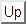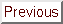Next: Relationship Between Self-Similarity and Up: Fractal Analysis Methods Previous: Mapping Real-World Time Series

## Detrended Fluctuation Analysis (DFA)

A simplified and general definition characterizes a time series as stationary if its mean, standard deviation and higher moments, as well as the correlation functions, are invariant under time translation. Signals that do not obey these conditions are nonstationary. As discussed above, a bounded time series can be mapped to a self-similar process by integration; for example, a sequence of coin flips can be mapped in this way to a one-dimensional random walk (see this tutorial for more on this subject, including hands-on experiments), a stationary integrated time series. Another challenge facing investigators applying this type of fractal analysis to physiologic time series, however, is that they are often highly nonstationary (Fig. 1a). The integration procedure will make the nonstationarity of the original data even more apparent.

To overcome this complication, we have introduced a modified root mean square analysis of a random walk, termed detrended fluctuation analysis ( DFA) [11, 12], which may be applied to the analysis of biological data. Among the advantages of DFA over conventional methods (e.g., spectral analysis and Hurst analysis) are that it permits the detection of intrinsic self-similarity embedded in a seemingly nonstationary time series, and also avoids the spurious detection of apparent self-similarity, which may be an artifact of extrinsic trends. This method has been successfully applied to a wide range of simulated and physiologic time series in recent years [11, 12, 13, 14, 15, 16].

Please note that although the DFA algorithm works well for certain types of nonstationary time series (especially slowly varying trends), it is not designed to handle all possible nonstationarities in real-world data.

To illustrate the DFA algorithm, we use the interbeat time series shown in Fig. 3a as an example. First, the interbeat interval time series (of total length N) is integrated,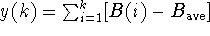, where B(i) is the i-th interbeat interval and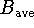is the average interbeat interval. As discussed above, this integration step maps the original time series to a self-similar process. Next we measure the vertical characteristic scale of the integrated time series. To do so, the integrated time series is divided into boxes of equal length, n. In each box of length n, a least squares line is fit to the data (representing the trend in that box) (Fig. 4). The y coordinate of the straight line segments is denoted by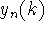. Next we detrend the integrated time series, y(k), by subtracting the local trend,, in each box. For a given box size n, the characteristic size of fluctuation for this integrated and detrended time series is calculated by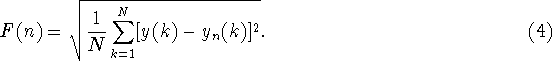(This quantity F is similar but not identical to the quantity s measured in the previous section.)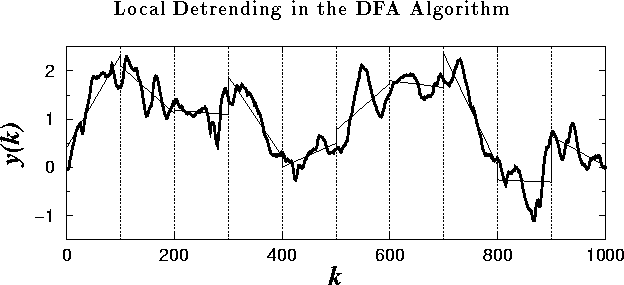Figure 4: The integrated time series:, where B(i) is the interbeat interval shown in Fig. 3(a). The vertical dotted lines indicate boxes of size n=100, and the solid straight line segments represent the ``trend'' estimated in each box by a linear least-squares fit.

This computation is repeated over all time scales (box sizes) to provide a relationship between F(n) and the box size n. Typically, F(n) will increase with box size n. A linear relationship on a double log graph indicates the presence of scaling (self-similarity)--the fluctuations in small boxes are related to the fluctuations in larger boxes in a power-law fashion. The slope of the line relating log F(n) to log n determines the scaling exponent (self-similarity parameter),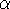, as discussed previously.Next: Relationship Between Self-Similarity and Up: Fractal Analysis Methods Previous: Mapping Real-World Time Series